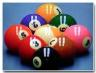## Tuesday, April 25, 2006

### 9 balls and only 2 weighingsYou are given 9 balls and they look exactly the same in color and physical appearance. You are also given a scale. To make things easy you are given that one of the balls amongst these 9 balls is heavy. With ONLY 2 weighings how can you find out which ball it is.

Level: EasyAditi Limaye said...

divide the 9 balls into 3 groups A,B,C
Weigh group A and B, either of them will be heavy,meaning the heaviest ball is in that group
else both will be equal, meaning the heaviest ball will be in C
Take any 2 balls in the heavier group and weigh them, the heavier of the 2 will be the heaviest ball, and if both are equal the ball left out from that group is the heaviest.

10:18 AM, April 25, 2006Kala Ramachandra said...

Separate the balls into grids of 3.

A) WEIGH ( 123 ) AGAINST ( 456 )
If equal go to step ( B )
If ( 123 ) Heavier go to step ( C )
If ( 456 ) Heavier go to step ( D )

B) WEIGH ( 7 ) AGAINST ( 8 )
If equal, BALL 9 is the HEAVY one
If ( 7 ) heavier, BALL 7 is the HEAVY one
If ( 8 ) heavier, BALL 8 is the HEAVY one

C) WEIGH ( 1 ) AGAINST ( 2 )
If equal, BALL 3 is the HEAVY one
If ( 1 ) heavier, BALL 1 is the HEAVY one
If ( 2 ) heavier, BALL 2 is the HEAVY one

D) WEIGH ( 4 ) AGAINST ( 5 )
If equal, BALL 6 is the HEAVY one
If ( 4 ) heavier, BALL 4 is the HEAVY one
If ( 5 ) heavier, BALL 5 is the HEAVY one

10:22 AM, April 25, 2006Karthik Nagaraj said...

Wow, Both Aditi and Kala have hit on the right spot.

10:25 AM, April 25, 2006